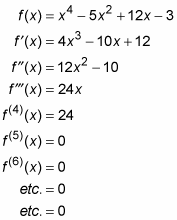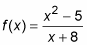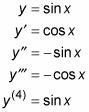##### Differential Equations Workbook For DummiesFinding a second, third, fourth, or higher derivative is incredibly simple. The second derivative of a function is just the derivative of its first derivative. The third derivative is the derivative of the second derivative, the fourth derivative is the derivative of the third, and so on.

For example, here’s a function and its first, second, third, and subsequent derivatives. In this example, all the derivatives are obtained by the power rule:All polynomial functions like this one eventually go to zero when you differentiate repeatedly. On the other hand, rational functions likeget messier and messier as you take higher and higher derivatives. And the higher derivatives of sine and cosine are cyclical. For example,The cycle repeats indefinitely with every multiple of four.

A first derivative tells you how fast a function is changing — how fast it’s going up or down — that’s its slope. A second derivative tells you how fast the first derivative is changing — or, in other words, how fast the slope is changing. A third derivative tells you how fast the second derivative is changing, which tells you how fast the rate of change of the slope is changing.

If you’re getting a bit lost here, don’t worry about it. It gets increasingly difficult to get a handle on what higher derivatives tell you as you go past the second derivative, because you start getting into a rate of change of a rate of change of a rate of change, and so on.